# Multiplication and Division of Decimals

Students use their knowledge of multiplication and division with whole numbers and with fractions to multiply and divide with decimals, and apply this understanding to the context of measurement conversion.

Math

Unit 6

## Unit Summary

In Unit 6, 5th grade students use their procedural knowledge of multiplication and division with whole numbers, combined with their newly acquired understanding of multiplication and division with fractions, to multiply and divide with decimals, reasoning about the placement of the decimal point. They then apply this to the context of word problems, including those involving measurement conversion.

In 4th grade, students were first introduced to decimal notation for fractions and reasoned about their size (4.NF.5—7). Then, in the first unit of 5th grade, students developed a deeper understanding of decimals as an extension of our place value system, understanding that the relationships of adjacent units apply to decimal numbers, as well (5.NBT.1), and using that understanding to compare, round, and represent decimals in various forms (5.NBT.2—4). Next, students learned to multiply and divide multi-digit whole numbers in Unit 2 (5.NBT.5—6), skills upon which decimal computations will rely. In Unit 4, students explored the other two operations with decimals not addressed in this unit: addition and subtraction (5.NBT.7). In Unit 5, students learned to multiply and divide with fractions, including relating fractions to the operation of division; multiplying a fraction by a fraction, including mixed numbers; and dividing a unit fraction by a whole number and vice versa (5.NF.3—7), which will help them make sense of analogous cases of decimal multiplication and division. Thus, this unit is dependent on a lot of prior learning, both in 4th grade and 5th grade.

This unit starts with multiplying a decimal by a single-digit whole number, then multiplying a decimal by a multi-digit whole number, and finally multiplying a decimal by another decimal. Then, students progress to dividing a decimal by a single-digit whole number, then dividing a decimal by a two-digit whole number, and finally solving cases involving decimal divisors. Throughout these topics, students use the same methods to compute decimal products and quotients as they did for whole-number products and quotients, but they must reason about the placement of the decimal point. It is only in the last lesson of each topic that students generalize the pattern of the placement of the decimal point. The various lines of reasoning, and their advantages and disadvantages, can be read on pages 19 and 20 of the NBT Progression linked in the “Unit-Specific Intellectual Preparation” section. Students also solve myriad word problems as well as write and solve expressions involving decimals as a way to support the major work (5.OA.1, 5.OA.2). Finally, the unit closes with students learning to convert among different-sized standard measurement units within a given measurement system and solve word problems that use those conversions (5.MD.1), which extends the work from 4th Grade Math of converting from a larger unit of measurement to a smaller one (4.MD.1—2). As noted in the Progressions, “this is an excellent opportunity to reinforce notions of place value for whole numbers and decimals, and the connection between fractions and decimals (e.g., $$2\tfrac{1}{2}$$ meters can be expressed as 2.5 meters or 250 centimeters)” (GM Progression, p. 26), as well as computations with these types of numbers (5.NBT.7, 5.NF), thus connecting the work of unit conversion with major work of the grade.

Reasoning about the placement of the decimal point affords students many opportunities to engage in mathematical practice, such as constructing viable arguments and critiquing the reasoning of others (MP.3) and looking for and expressing regularity in repeated reasoning (MP.8). For example, “students can summarize the results of their reasoning as specific numerical patterns and then as one general overall pattern such as ‘the number of decimal places in the product is the sum of the number of decimal places in each factor’” (NBT Progression, p. 20).

In 6th Grade Math, students will become fluent with all decimals computations that they’ve developed in 5th grade (6.NS.3). In 7th Grade Math, students will also learn that every fraction can be represented with a decimal that either terminates or repeats. Then in 8th Grade Math, students learn that terminating and repeating decimals are rational numbers and that there are numbers that are irrational whose decimal expansion does not repeat. Then, students use the work they start in this unit in 8th grade in the context of scientific notation. Thus, this unit has many interesting connections and applications for many years to come.

Pacing: 27 instructional days (24 lessons, 2 flex days, 1 assessment day)

Fishtank Plus for Math

Unlock features to optimize your prep time, plan engaging lessons, and monitor student progress.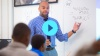## Assessment

The following assessments accompany Unit 6.

### Pre-Unit

Have students complete the Pre-Unit Assessment and Pre-Unit Student Self-Assessment before starting the unit. Use the Pre-Unit Assessment Analysis Guide to identify gaps in foundational understanding and map out a plan for learning acceleration throughout the unit.

### Mid-Unit

Have students complete the Mid-Unit Assessment after lesson 16.

### Post-Unit

Use the resources below to assess student understanding of the unit content and action plan for future units.

Expanded Assessment Package

Use student data to drive your planning with an expanded suite of unit assessments to help gauge students’ facility with foundational skills and concepts, as well as their progress with unit content.

## Unit Prep

### Intellectual Prep

#### Intellectual Prep for All Units

• Read and annotate “Unit Summary” and “Essential Understandings” portion of the unit plan.
• Do all the Target Tasks and annotate them with the “Unit Summary” and “Essential Understandings” in mind.
• Take the Post-Unit Assessment.

#### Unit-Specific Intellectual Prep

 Area model for multiplication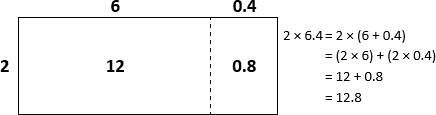Partial products algorithm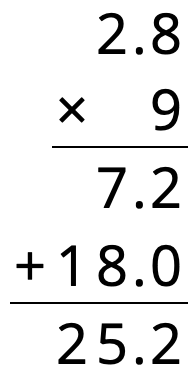Standard algorithm for multiplication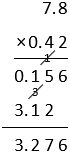Area model for division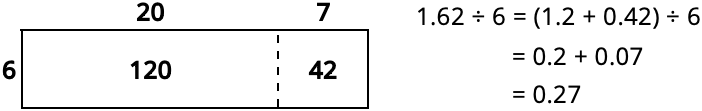Partial quotients algorithm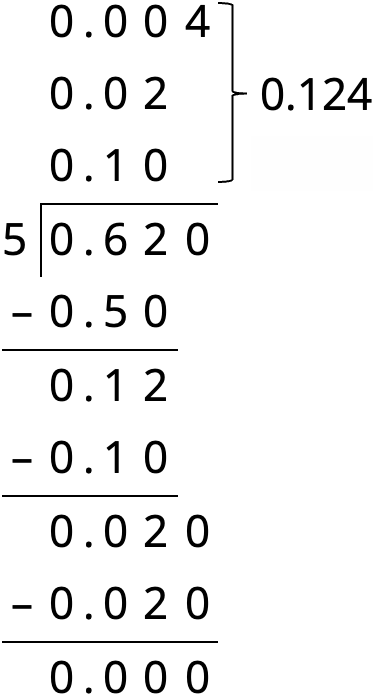### Essential Understandings

• General methods used for computing products and quotients of whole numbers extend to products and quotients of decimals, with the additional issue of placing a decimal point in the solution. There are several lines of reasoning that students can use to explain the placement of the decimal point in products and quotients of decimals.
• Students may use estimation to assess the reasonableness of their solution. As with whole-number computation, some computational estimates can be better than others, depending on what numbers are chosen to use in place of the actual values. Using estimation to place the decimal point, however, isn’t always a reliable strategy, especially in cases they will see in later grades such as $${0.0043 \times 0.00078}$$.

### Vocabulary

conversion factor

To see all the vocabulary for Unit 6, view our 5th Grade Vocabulary Glossary.

## Unit Practice

Word Problems and Fluency Activities

Access daily word problem practice and our content-aligned fluency activities created to help students strengthen their application and fluency skills.## Lesson Map

Topic A: Multiplying Decimals

Topic B: Dividing Decimals

Topic C: Decimal Expressions and Real-World Problems

Topic D: Measurement Conversion and Real-World Problems

## Common Core Standards

Key

Major Cluster

Supporting Cluster

### Core Standards

#### Measurement and Data

• 5.MD.A.1 — Convert among different-sized standard measurement units within a given measurement system (e.g., convert 5 cm to 0.05 m), and use these conversions in solving multi-step, real world problems.

#### Number and Operations in Base Ten

• 5.NBT.B.7 — Add, subtract, multiply, and divide decimals to hundredths, using concrete models or drawings and strategies based on place value, properties of operations, and/or the relationship between addition and subtraction; relate the strategy to a written method and explain the reasoning used.

#### Operations and Algebraic Thinking

• 5.OA.A.1 — Use parentheses, brackets, or braces in numerical expressions, and evaluate expressions with these symbols.
• 5.OA.A.2 — Write simple expressions that record calculations with numbers, and interpret numerical expressions without evaluating them. For example, express the calculation "add 8 and 7, then multiply by 2" as 2 × (8 + 7). Recognize that 3 × (18932 + 921) is three times as large as 18932 + 921, without having to calculate the indicated sum or product.

• 4.MD.A.1
• 4.MD.A.2

• 5.NBT.A.1
• 5.NBT.A.2
• 5.NBT.A.4
• 5.NBT.B.5
• 5.NBT.B.6

• 5.NF.B.3
• 5.NF.B.4
• 5.NF.B.7

• 6.EE.A.2
• 6.EE.A.3
• 6.EE.A.4

• 6.NS.B.3
• 6.NS.B.4

### Standards for Mathematical Practice

• CCSS.MATH.PRACTICE.MP1 — Make sense of problems and persevere in solving them.

• CCSS.MATH.PRACTICE.MP2 — Reason abstractly and quantitatively.

• CCSS.MATH.PRACTICE.MP3 — Construct viable arguments and critique the reasoning of others.

• CCSS.MATH.PRACTICE.MP4 — Model with mathematics.

• CCSS.MATH.PRACTICE.MP5 — Use appropriate tools strategically.

• CCSS.MATH.PRACTICE.MP6 — Attend to precision.

• CCSS.MATH.PRACTICE.MP7 — Look for and make use of structure.

• CCSS.MATH.PRACTICE.MP8 — Look for and express regularity in repeated reasoning.

Unit 5

Multiplication and Division of Fractions

Unit 7

Patterns and the Coordinate Plane

## Request a Demo

See all of the features of Fishtank in action and begin the conversation about adoption.

Yes

No